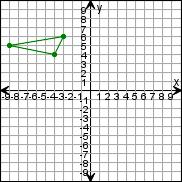Create a new printableGeometry
Math Worksheets

Sample - Click above to make a new math worksheet (PDF).
 Name _____________________________Date ___________________
Transformations
Draw the triangle after the transformations.

 1 * This is a pre-made sheet.Use the link at the top of the page for a printable page.
 2 Translate the triangle 3 units down.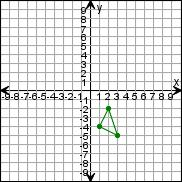3 Translate the triangle left 2 units, then 4 units up.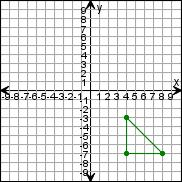4 Translate the triangle down 1 unit, then 1 unit left.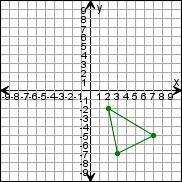5 Translate the triangle up 5 units, then 1 unit right.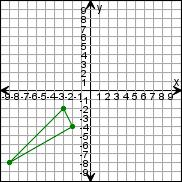6 Translate the triangle down 5 units, then 4 units left.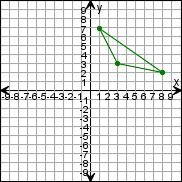7 Translate the triangle right 4 units, then 5 units down.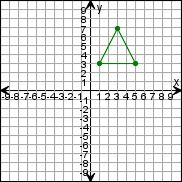8 Translate the triangle right 6 units, then 2 units up.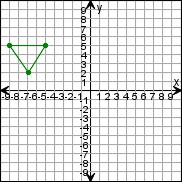9 Translate the triangle 1 unit right.